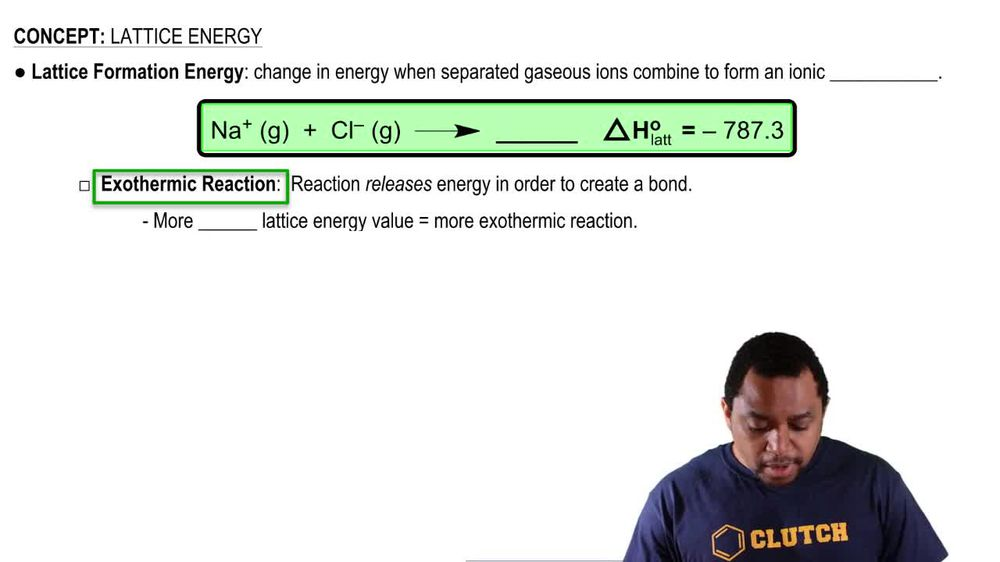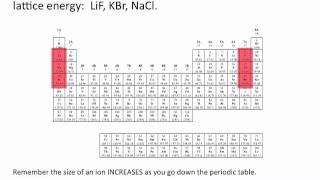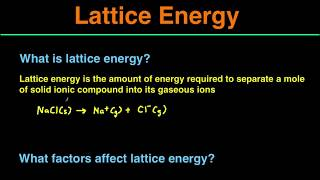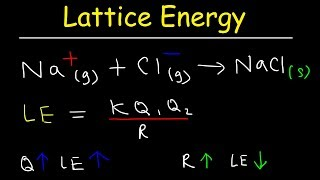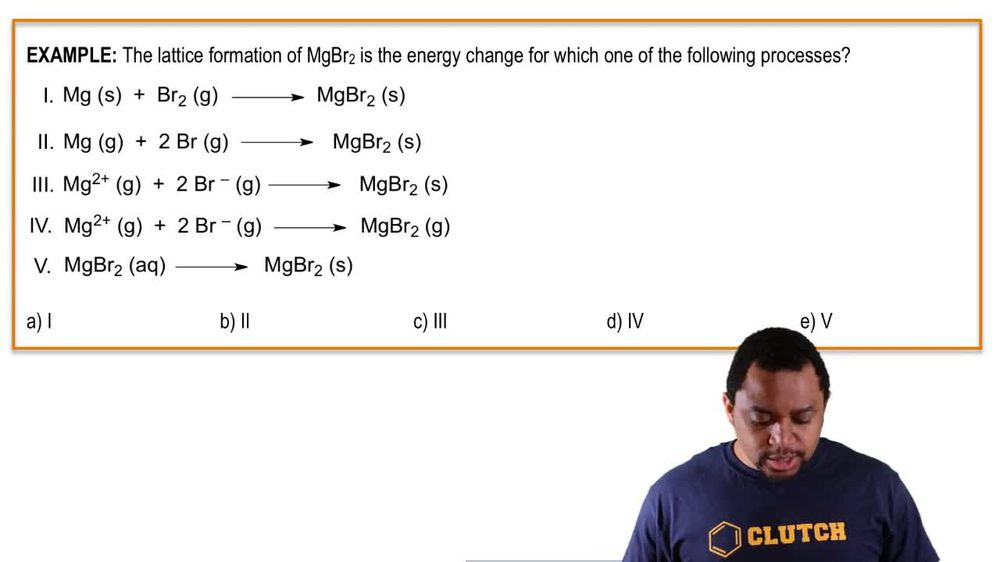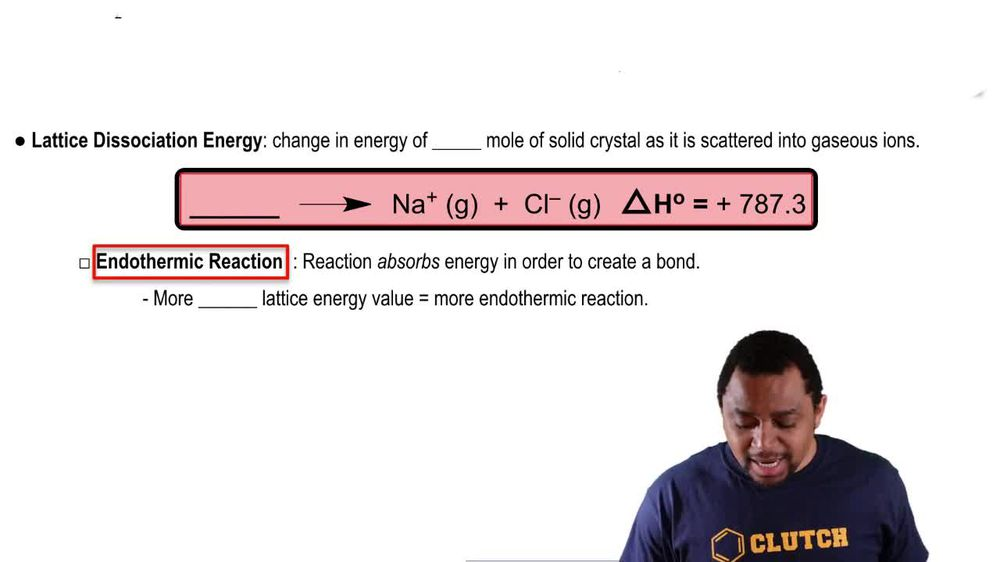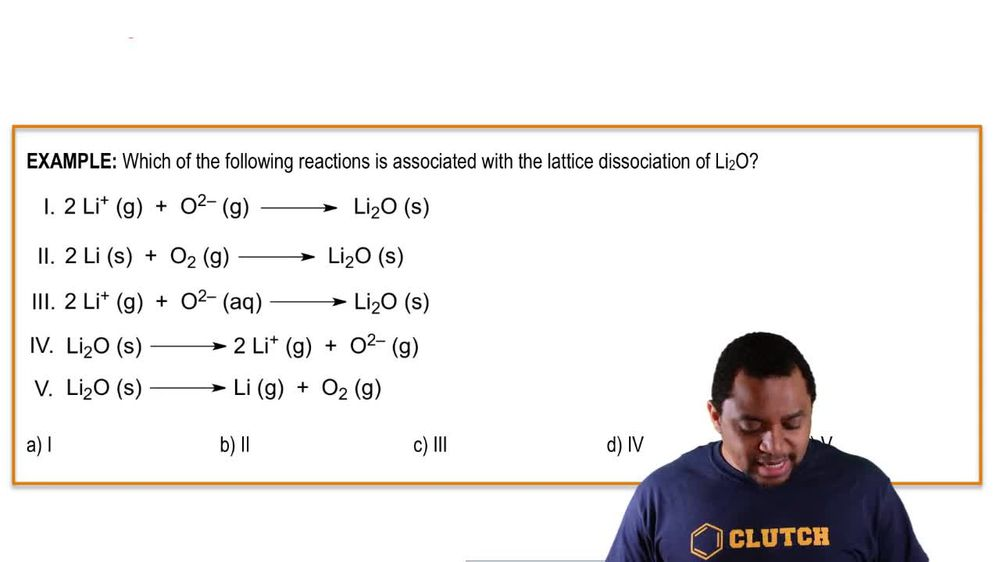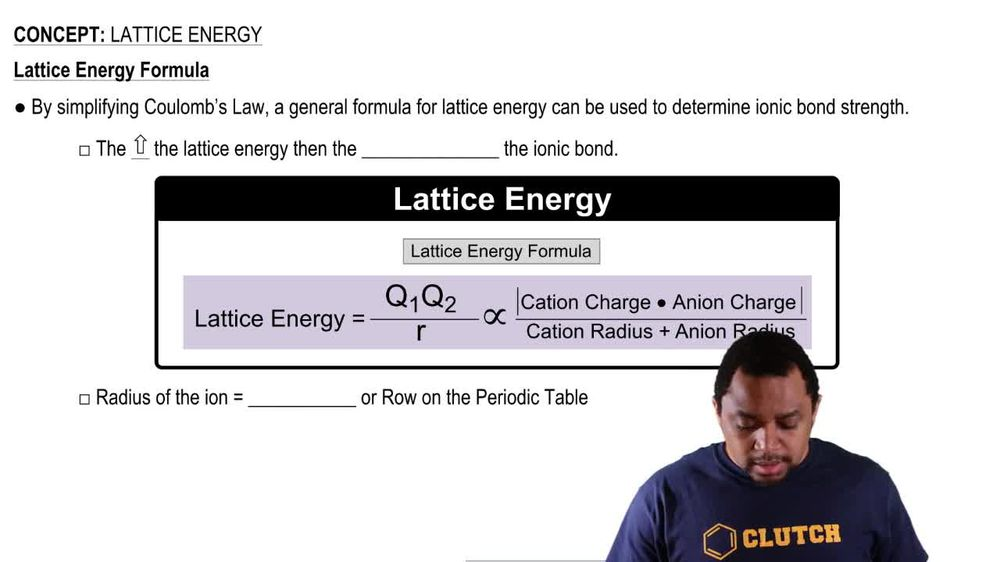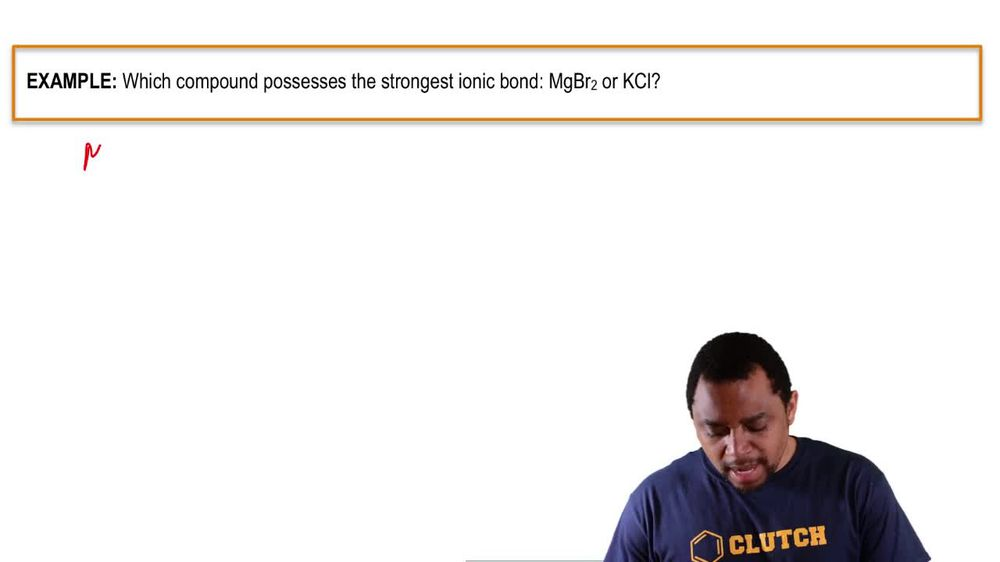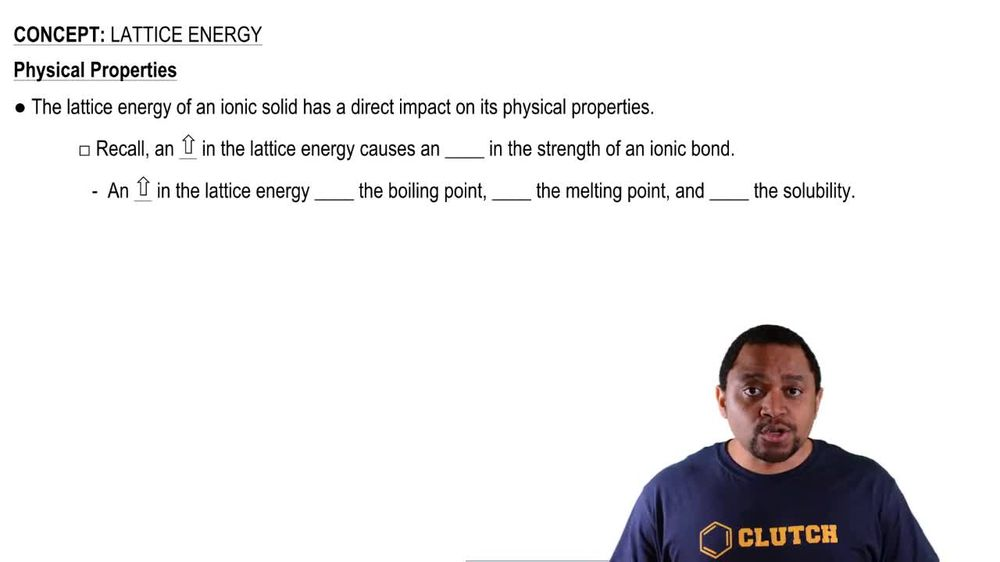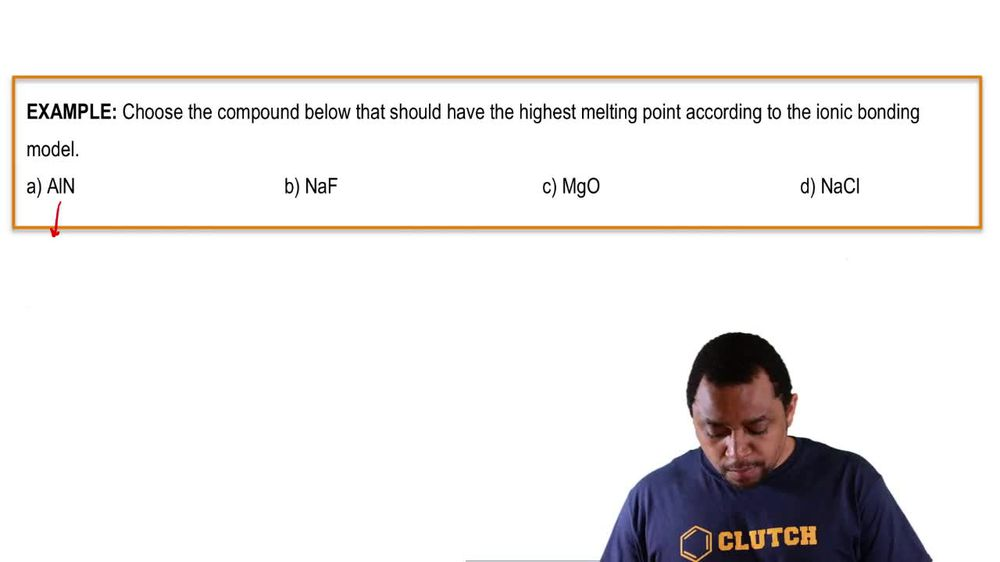Start typing, then use the up and down arrows to select an option from the list.
1. 11. Bonding & Molecular Structure2. Lattice Energy# Lattice Energy Example 3

by Jules Bruno
79 views
1
0
So here it's saying which compound possesses the strongest Ionic bond? We have magnesium bromide versus potassium chloride. So for magnesium bromide, we break it up into its ions. So that breaks up into magnesium two plus NPR minus one for their lattice energy. Remember, it's their charges in absolute terms. So plus two times negative one divided by their period numbers added together. So magnesium, if you look on the periodic table is in row three. Brahman is in row four. So that is to substance for its lattice energy. Potassium chloride made up of potassium ion and chloride ion look and see where they are on the periodic table. So remember, we multiply their charges in absolute terms. Now look on the periodic table. Potassium is in the fourth row of the periodic table, so period for chlorine is in the third row. So here lattice energy equals 1/7. So if you compare the two, we see that magnesium bromide has a larger lattice energy, and therefore it has a stronger ionic bond. Remember, the stronger your your lattice energy or high your lattice energy, and the stronger your Ionic bond will be